# Series- Test 4

1)

Find the missing figure in the series from the given answer figures.

[SSC CML, 1999]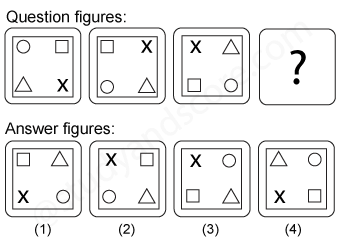2)

Find the missing figure in the series from the given answer figures.

[SSC CML, 1999]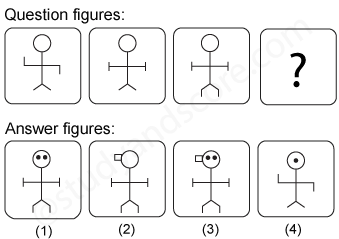3)

Find the missing figure in the series from the given answer figures.

[SSC CML, 1999]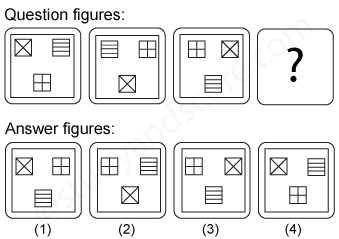4)

Find the missing figure in the series from the given answer figures.

[SSC CML, 1999]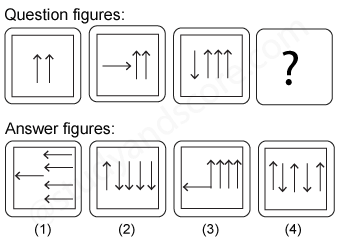5)

A series is given with a figure missing in it. Choose the correct alternative from the given options to complete the series.

[SSC CML, 2000]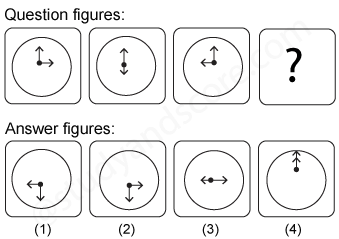--Share with your friends --